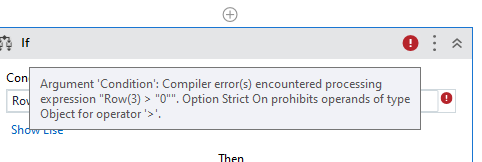# How to use For Each in DataTable

Hi All,

I have read this using Read Range, Output as DT and now I want the Team Name (first column) wherever the value is greater than zero in Total column (Last column)

@Chetan_Wagh

If you want to filter and get only greater than zero…use a filter datatable first and add the condition

And then use for each row in datatable activity

Or …you can as well use a if condition inside your for loop to seggreate greater than zero values

`Currentrow("ColumnName").ToString `will give the data of each column value for each row inside the for loop

Hope this helps

Cheers

1 Like

I Tried with For each row in DT but getting the following errorUse filter datatable activity, pass the column name and set the value as 0

or use the below linq query

``````(From r In dtInput.AsEnumerable
Where CInt(r("Team Name").ToString().Trim()) > 0
Select d = r).CopyToDataTable
``````

Regards!

1 Like

@Chetan_Wagh

Remove the inverted comma around zero… numbers dont need inverted comma

`Cint(Row(3).ToString) > 0`

Cheers

1 Like

Thank you again for the quick help @Anil_G .1 Like

Can you describe the If condition.

LMK if this is correct. IF, Row(“ColumnIndex”)>0

Ohhh.Thanks a lot

1 Like

@Chetan_Wagh

`If(IsNumeric(Row(3).ToString),Cint(Row(3).ToString),0)>0`